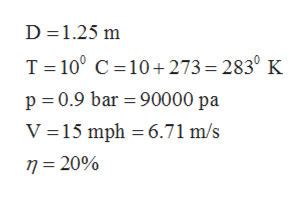# Fluid Mechanics : Energy and Power:An engineer is considering the development of a small wind turbine (D=1.25m) for home applications. The design wind speed is 15 mph(6.706m/s) at 10oC and 0.9 bar (90kPa). The efficiency of the turbine is 20%, that is 20% of the kinetic energy of the wind can be extracted. Estimate the power in watts that can be produced by the turbine.Hints:1) In the time interval delta t, the amount of mass that flows through the rotor is:    delta m = mdot delta t 2) The kinetic energy in the flow is:    (delta m V2/2)

Question
28 views

Fluid Mechanics : Energy and Power:

An engineer is considering the development of a small wind turbine (D=1.25m) for home applications. The design wind speed is 15 mph(6.706m/s) at 10oC and 0.9 bar (90kPa). The efficiency of the turbine is 20%, that is 20% of the kinetic energy of the wind can be extracted. Estimate the power in watts that can be produced by the turbine.

Hints:

1) In the time interval delta t, the amount of mass that flows through the rotor is:    delta m = mdot delta t

2) The kinetic energy in the flow is:    (delta m V2/2)

check_circle

Step 1

Given Information:help_outlineImage TranscriptioncloseD 1.25 m T 100 C 10+273 = 283° K p 0.9 bar 90000 pa V 15 mph 6.71 m/s n- 20% fullscreen
Step 2

We will have to find density using Ideal Gas Law:

Step 3

Now we will find the area ...

### Want to see the full answer?

See Solution

#### Want to see this answer and more?

Solutions are written by subject experts who are available 24/7. Questions are typically answered within 1 hour.*

See Solution
*Response times may vary by subject and question.
Tagged in

### Civil Engineering